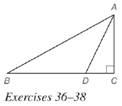Chapter 5.6, Problem 38EElementary Geometry For College St...

7th Edition
Alexander + 2 others
ISBN: 9781337614085

Solutions

Chapter
SectionElementary Geometry For College St...

7th Edition
Alexander + 2 others
ISBN: 9781337614085
Textbook Problem

In right Δ A B C with right ∠ C , A D → bisects ∠ B A C . If A C = 6 and D C = 3 , find B D and A B . (HINT: Let B D = x and A B = 2 x . Then use the Pythagorean Theorem.)To determine

To find:

BD and AB.

Explanation

Given:

In a right ΔABC with right C, AD bisects BAC and AC=6,DC=3.

Theorem used:

Angle bisector theorem:

If a ray bisects one angle of a triangle, then it divides the opposite side into segments whose lengths are proportional to the lengths of the two sides that form the bisected angle.

Calculation:

Consider a right ΔABC.

Given that AD bisects BAC.

By angle bisector theorem, we get

ABAC=BDDC

ACDC=ABBD

We have AC=6,DC=3.

63=ABBD21=ABBD

AB:BD=2:1

Let AB=2x,BD=x

From the figure we see that, BC=BD+DC

BC=x+3

Consider the right triangle ABC with right angle at C

Still sussing out bartleby?

Check out a sample textbook solution.

See a sample solution

The Solution to Your Study Problems

Bartleby provides explanations to thousands of textbook problems written by our experts, many with advanced degrees!

Get Started

Differentiate. G(x)=x222x+1

Single Variable Calculus: Early Transcendentals, Volume I

Fill in each blank: 88oz=lb

Elementary Technical Mathematics

Convert the expressions in Exercises 8596 radical form. 0.2x2/3+37x1/2

Finite Mathematics and Applied Calculus (MindTap Course List)

Differentiate. y = sin cos

Single Variable Calculus: Early Transcendentals

True or False: is a rational function.

Study Guide for Stewart's Single Variable Calculus: Early Transcendentals, 8th